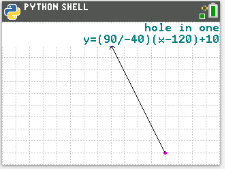# Activities

•6-8
9-12

• ##### Device
•TI-84 Plus CE Python

## Putt Putt with Python#### Activity Overview

In this coding activity for the TI-84 Plus CE Python edition, students will create a golf game.  The golf game gives the user two random points, one for the golf ball and one for the golf hole.  The user will enter a value for the slope.

#### Objectives

• Use the input function and a variable to collect and store data from a user
• Use the randint() function to generate random integers
• Use the plot library to plot points
• Use the plot library to plot a line segment
•  Use the point slope form of a line to plot a line segment